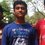[Multinomial Theorem] Combinatorics Question

I know how to solve this question using a very long and tedious method. However, my friend says that he solved it using Multinomial Theorem. I already know the binomial theorem but I wish to know how to solve this question using Multinomial Theorem. Please help!!!

Find the number of words that can be formed taking 6 letters of the word ALLAHABAD at a time.Note by Rohan Rao
6 years, 9 months ago

This discussion board is a place to discuss our Daily Challenges and the math and science related to those challenges. Explanations are more than just a solution — they should explain the steps and thinking strategies that you used to obtain the solution. Comments should further the discussion of math and science.

When posting on Brilliant:

• Use the emojis to react to an explanation, whether you're congratulating a job well done , or just really confused .
• Ask specific questions about the challenge or the steps in somebody's explanation. Well-posed questions can add a lot to the discussion, but posting "I don't understand!" doesn't help anyone.
• Try to contribute something new to the discussion, whether it is an extension, generalization or other idea related to the challenge.
• Stay on topic — we're all here to learn more about math and science, not to hear about your favorite get-rich-quick scheme or current world events.

MarkdownAppears as
*italics* or _italics_ italics
**bold** or __bold__ bold

- bulleted
- list

• bulleted
• list

1. numbered
2. list

1. numbered
2. list
Note: you must add a full line of space before and after lists for them to show up correctly
paragraph 1

paragraph 2

paragraph 1

paragraph 2

> This is a quote
This is a quote
# I indented these lines
# 4 spaces, and now they show
# up as a code block.

print "hello world"
# I indented these lines
# 4 spaces, and now they show
# up as a code block.

print "hello world"
MathAppears as
Remember to wrap math in $$...$$ or $...$ to ensure proper formatting.
2 \times 3 $2 \times 3$
2^{34} $2^{34}$
a_{i-1} $a_{i-1}$
\frac{2}{3} $\frac{2}{3}$
\sqrt{2} $\sqrt{2}$
\sum_{i=1}^3 $\sum_{i=1}^3$
\sin \theta $\sin \theta$
\boxed{123} $\boxed{123}$

Sort by:

it can be solved by using permutations you don't even need using some other theorems

- 6 years, 9 months ago

I already know how to solve it using permutations, but the Multinomial theorem is supposed to make it much shorter.

- 6 years, 9 months ago

The multinomial theorem is a generalization of the binomial theorem. It is proved in a way similar to the binomial theorem, so if you know the proof of the binomial theorem, you should understand the multinomial theorem. You can find further information here and here.

In regards to your problem, we could consider a generating function approach. Consider the expansion of $(A + L + H + B + D)^{6}$. We are looking for the sum of the coefficients of the terms of the form $A^{a}L^{l}H^{h}B^{b}D^{d}$, where $a \leq 4$, $l \leq 2$, and $h, b, d \leq 1$ (these restrictions come from the number of times each letter appears in ALLAHABAD). We can use the multinomial theorem to find the coefficients of each of these terms. I have not actually worked out this problem, but it seems that this solution still requires a bit of calculation.

- 6 years, 9 months ago

With regards to the generating function approach, the setup indicates that you rather consider the expansion of $(1+x+x^2+x^3+x^4)(1+x+x^2)(1+x)(1+x)(1+x)$ and calculate the coefficient of $x^6$.

Using the multinomial theorem greatly over counts, and there is no easy way to remove the over counting. PIE comes to mind, but there are too many cases.

Staff - 6 years, 9 months ago

What I posted wasn't entirely true. As you're allowed to rearrange the terms, you want to look at the exponential generating function instead. In this case, we're interested in the coefficient of $x^6$ in

$6! (1 + \frac {1}{1!}x + \frac {1}{2!} x^2 + \frac {1}{3!}x^3 + \frac {1}{4!}x^4)(1 + \frac {1}{1!}x + \frac {1}{2!}x^2)(1+\frac {1}{1!} x ) ( 1 + \frac {1}{1!}x) ( 1 + \frac {1}{1!}x)$

Staff - 6 years, 8 months ago

Do you know the answer? I'm getting 2115. If I'm correct then I can probably guide you.

- 6 years, 9 months ago

The answer is correct. Please tell me how to do it using the generating function approach Calvin and Omid mentioned above. Thanks!

- 6 years, 9 months ago

How much do you understand of generating functions? For example, do you know how to create the generating function that will yield the number of heads in 10 coin tosses?

Staff - 6 years, 8 months ago

I don't know what they are. Please explain.

- 6 years, 8 months ago

Can you state the Multinomial Theorem, or what you understand of it?

Staff - 6 years, 9 months ago

I didn't understand the Multinomial Theorem at all, since the way my friend explained it was confusing. Please direct me to the right website with some solved example questions so I can understand it better. Also tell me how to solve the question I have posted above. Thank you!

- 6 years, 9 months ago

The multinomial theorem is a pretty direct extension of the binomial theorem. Before I talk about that, let's see if you understand how to apply the binomial theorem first.

Let's say I have 4 A's and 6 L's. How many 5-letter 'words' can I form? How would you solve this using only the Binomial theorem?

Staff - 6 years, 8 months ago

The number of words should be the coefficient of x^{5} in (1+x^2 + x^3 + x^4 +x^5)(1+x+x^2+x^3+x^4)

- 6 years, 8 months ago